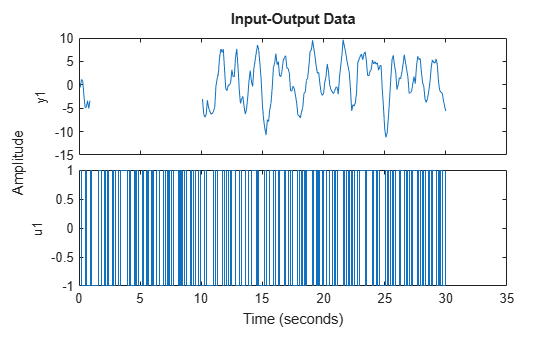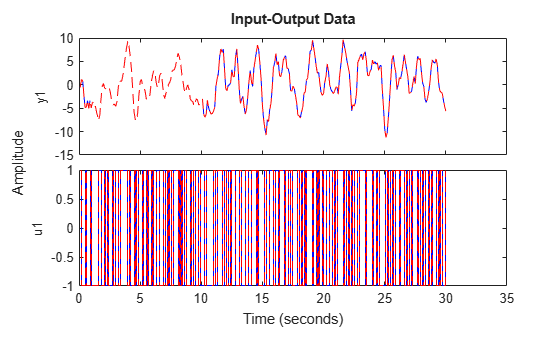# misdata

Reconstruct missing input and output data

## Syntax

```Datae = misdata(Data) Datae = misdata(Data,Model) Datae = misdata(Data,MaxIterations,Tol) Datae = misdata(___,Name,Value) ```

## Description

`Datae = misdata(Data)` reconstructs missing input and output time-domain data.

`Data` contains time-domain data, which can be in the form of a `timetable`, a comma-separated pair of numeric matrices, or a time-domain `iddata` object.

Missing data samples (both in inputs and in outputs) are expressed as `NaN`s. `Datae`, which is the same form as `Data`, contains the reconstructed data set where the missing data has been replaced by reasonable estimates.

`Datae = misdata(Data,Model)` specifies a model used for the reconstruction of missing data. `Model` is any linear identified model (`idtf`, `idproc`, `idgrey`, `idpoly`, `idss`). If no suitable model is known, it is estimated in an iterative fashion using default order state-space models.

`Datae = misdata(Data,MaxIterations,Tol)` specifies maximum number of iterations and tolerance. `MaxIterations` is the maximum number of iterations carried out (the default is 10). The iterations are terminated when the difference between two consecutive data estimates differs by less than `Tol`%. The default value of `Tol` is `1`.

`Datae = misdata(___,Name,Value)` uses additional model options specified by one or more name-value arguments.

The available arguments consist of:

• `InputName` and `OutputName` — Input and output channel names. Use these arguments especially when `data` is a timetable that has more variables than you want to use, or when there. Also use `OutputName` to identify the output channels when the timetable `data` contains more than one output variable. Otherwise, the software interprets only the last variable as an output channel.

For example, specify the input and output signal variable names using ```Datae = misdata(data,'InputName',["u1","u3"],'OutputName',"y1")```. You can use this syntax with any of the previous input-argument combinations.

• `Ts` and `TimeUnit` — Sample time and time units. Use these arguments especially when you are `data` contains numeric matrices, which do not provide sample-time information.

## Examples

collapse all

Load data with missing data points.

`load('missing_data.mat')`

`missing_data` is an `iddata` object containing input-output data.

Plot the data.

`plot(missing_data)`The output data contains missing data between indices 10 and 100.

To reconstruct missing data using a specified model, estimate the model using measured data that has no missing samples. In this example, estimate a transfer function model with 2 poles.

```data2 = missing_data(101:end); model = tfest(data2,2);```

Reconstruct the missing data.

`datae = misdata(missing_data,model);`

Plot the original and reconstructed data.

`plot(missing_data,'b',datae,'--r')`If you do not specify a model for reconstructing the data, the software alternates between estimating missing data and estimating models, based on the current data reconstruction.

## Algorithms

For a given model, the missing data is estimated as parameters so as to minimize the output prediction errors obtained from the reconstructed data. See Section 14.2 in Ljung (1999). Treating missing outputs as parameters is not the best approach from a statistical point of view, but is a good approximation in many cases.

When no model is given, the algorithm alternates between estimating missing data and estimating models, based on the current reconstruction.

## Version History

Introduced before R2006a

expand all